# Measures of Association

Go back to Tutorial

An association is any relationship between two measured quantities that renders them statistically dependent. The term “association” is closely related to the term “correlation.” Both terms imply that two or more variables vary according to some pattern. However, correlation is more rigidly defined by some correlation coefficient which measures the degree to which the association of the variables tends to a certain pattern.

It provides information about the relatedness between variables so as to help estimate the existence of a relationship between variables and its strength.

Covariance

Variables are positively related if they move in the same direction. Variables are inversely related if they move in opposite directions.

The relationship between two variables can be illustrated in a graph. The graph indicates that as economic growth increases, stock market returns also increase. The graph on the right is an example of how the inverse relationship between oil production and gasoline prices might appear. It illustrates that as oil production increases, gas prices fall.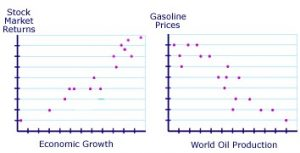Covariance shows how the variable y reacts to a variation of the variable x. Its formula is for a population cov( X, Y ) = ∑( xi − µx) (yi − µy) / N

Formula for sample is as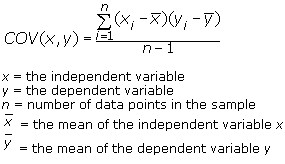Consider the table below, which describes the rate of economic growth (xi) and the rate of return on the S&P 500 (yi).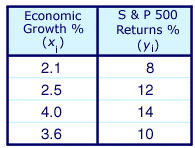Using the covariance formula, you can determine whether economic growth and S&P 500 returns have a positive or inverse relationship. Before you compute the covariance, calculate the mean of x and y.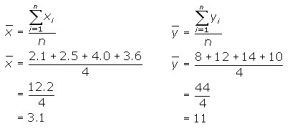Now you can identify the variables for the covariance formula as follows.

x = 2.1, 2.5, 4.0, and 3.6 (economic growth)

y = 8, 12, 14, and 10 (S&P 500 returns)

= 3.1

= 11

Substitute these values into the covariance formula to determine the relationship between economic growth and S&P 500 returns.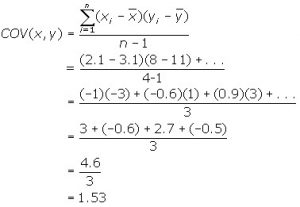The covariance between the returns of the S&P 500 and economic growth is 1.53. Since the covariance is positive, the variables are positively related—they move together in the same direction.

Correlation coefficient (r)

Pearson’s correlation coefficient is the covariance of the two variables divided by the product of their standard deviations.

The quantity r, called the linear correlation coefficient, measures the strength and the direction of a linear relationship between two variables. The linear correlation coefficient is sometimes referred to as the Pearson product moment correlation coefficient in honor of its developer Karl Pearson. The mathematical formula for computing r is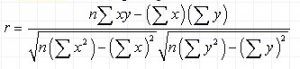where n is the number of pairs of data.

The value of r is such that -1 < r < +1. The + and – signs are used for positive linear correlations and negative linear correlations, respectively.

• Positive correlation: If x and y have a strong positive linear correlation, r is close to +1. An r value of exactly +1 indicates a perfect positive fit. Positive values indicate a relationship between x and y variables such that as values for x increases, values for y also increase.
• Negative correlation: If x and y have a strong negative linear correlation, r is close to -1. An r value of exactly -1 indicates a perfect negative fit. Negative values indicate a relationship between x and y such that as values for x increase, values for y decrease.
• No correlation: If there is no linear correlation or a weak linear correlation, r is close to 0. A value near zero means that there is a random, nonlinear relationship between the two variables
• A perfect correlation of ± 1 occurs only when the data points all lie exactly on a straight line. If r = +1, the slope of this line is positive. If r = -1, the slope of this line is negative.

A correlation greater than 0.8 is generally described as strong, whereas a correlation less than 0.5 is generally described as weak. These values can vary based upon the “type” of data being examined.

Certified Inventory and Warehouse Analytics Professional

Go back to Tutorial